# Difference between revisions of "Directory:Jon Awbrey/Papers/Riffs and Rotes"

## Idea

Let $$\text{p}_i\!$$ be the $$i^\text{th}\!$$ prime, where the positive integer $$i\!$$ is called the index of the prime $$\text{p}_i\!$$ and the indices are taken in such a way that $$\text{p}_1 = 2.\!$$ Thus the sequence of primes begins as follows:

 $$\begin{matrix} \text{p}_1 = 2, & \text{p}_2 = 3, & \text{p}_3 = 5, & \text{p}_4 = 7, & \text{p}_5 = 11, & \text{p}_6 = 13, & \text{p}_7 = 17, & \text{p}_8 = 19, & \ldots \end{matrix}$$

The prime factorization of a positive integer $$n\!$$ can be written in the following form:

 $$n ~=~ \prod_{k = 1}^{\ell} \text{p}_{i(k)}^{j(k)},\!$$

where $$\text{p}_{i(k)}^{j(k)}\!$$ is the $$k^\text{th}\!$$ prime power in the factorization and $$\ell\!$$ is the number of distinct prime factors dividing $$n.\!$$ The factorization of $$1\!$$ is defined as $$1\!$$ in accord with the convention that an empty product is equal to $$1.\!$$

Let $$I(n)\!$$ be the set of indices of primes that divide $$n\!$$ and let $$j(i, n)\!$$ be the number of times that $$\text{p}_i\!$$ divides $$n.\!$$ Then the prime factorization of $$n\!$$ can be written in the following alternative form:

 $$n ~=~ \prod_{i \in I(n)} \text{p}_{i}^{j(i, n)}.\!$$

For example:

 $$\begin{matrix} 123456789 & = & 3^2 \cdot 3607 \cdot 3803 & = & \text{p}_2^2 \text{p}_{504}^1 \text{p}_{529}^1. \end{matrix}$$

Each index $$i\!$$ and exponent $$j\!$$ appearing in the prime factorization of a positive integer $$n\!$$ is itself a positive integer, and thus has a prime factorization of its own.

Continuing with the same example, the index $$504\!$$ has the factorization $$2^3 \cdot 3^2 \cdot 7 = \text{p}_1^3 \text{p}_2^2 \text{p}_4^1\!$$ and the index $$529\!$$ has the factorization $${23}^2 = \text{p}_9^2.\!$$ Taking this information together with previously known factorizations allows the following replacements to be made in the above expression:

 \begin{array}{rcl} 2 & \mapsto & \text{p}_1^1 \'"UNIQ-MathJax1-QINU"' '"UNIQ-MathJax2-QINU"' '"UNIQ-MathJax3-QINU"' '"UNIQ-MathJax4-QINU"' :{| border="1" cellpadding="20" | [[Image:Rote 802701 Big.jpg|330px]] |} '"UNIQ-MathJax5-QINU"' {| align="center" border="1" cellpadding="6" |+ style="height:25px" | \(a(n) = \text{Rote Height of}~ n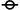$$1\!$$ $$a(1) ~=~ 0$$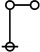$$\text{p}\!$$ $$a(2) ~=~ 1$$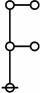$$\text{p}_\text{p}\!$$ $$a(3) ~=~ 2$$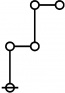$$\text{p}^\text{p}\!$$ $$a(4) ~=~ 2$$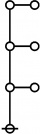$$\text{p}_{\text{p}_\text{p}}\!$$ $$a(5) ~=~ 3$$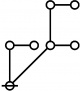$$\text{p} \text{p}_\text{p}\!$$ $$a(6) ~=~ 2$$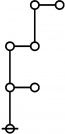$$\text{p}_{\text{p}^\text{p}}\!$$ $$a(7) ~=~ 3$$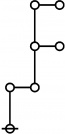$$\text{p}^{\text{p}_\text{p}}\!$$ $$a(8) ~=~ 3$$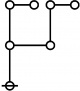$$\text{p}_\text{p}^\text{p}\!$$ $$a(9) ~=~ 2$$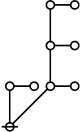$$\text{p} \text{p}_{\text{p}_\text{p}}\!$$ $$a(10) ~=~ 3$$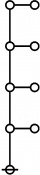$$\text{p}_{\text{p}_{\text{p}_\text{p}}}\!$$ $$a(11) ~=~ 4$$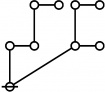$$\text{p}^\text{p} \text{p}_\text{p}\!$$ $$a(12) ~=~ 2$$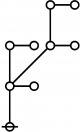$$\text{p}_{\text{p} \text{p}_\text{p}}\!$$ $$a(13) ~=~ 3$$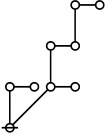$$\text{p} \text{p}_{\text{p}^\text{p}}\!$$ $$a(14) ~=~ 3$$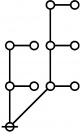$$\text{p}_\text{p} \text{p}_{\text{p}_\text{p}}\!$$ $$a(15) ~=~ 3$$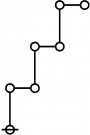$$\text{p}^{\text{p}^\text{p}}\!$$ $$a(16) ~=~ 3$$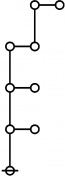$$\text{p}_{\text{p}_{\text{p}^\text{p}}}\!$$ $$a(17) ~=~ 4$$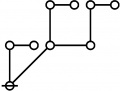$$\text{p} \text{p}_\text{p}^\text{p}\!$$ $$a(18) ~=~ 2$$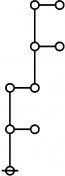$$\text{p}_{\text{p}^{\text{p}_\text{p}}}\!$$ $$a(19) ~=~ 4$$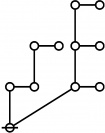$$\text{p}^\text{p} \text{p}_{\text{p}_\text{p}}\!$$ $$a(20) ~=~ 3$$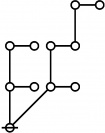$$\text{p}_\text{p} \text{p}_{\text{p}^\text{p}}\!$$ $$a(21) ~=~ 3$$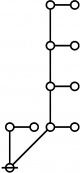$$\text{p} \text{p}_{\text{p}_{\text{p}_\text{p}}}\!$$ $$a(22) ~=~ 4$$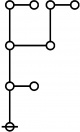$$\text{p}_{\text{p}_\text{p}^\text{p}}\!$$ $$a(23) ~=~ 3$$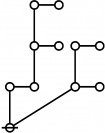$$\text{p}^{\text{p}_\text{p}} \text{p}_\text{p}\!$$ $$a(24) ~=~ 3$$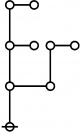$$\text{p}_{\text{p}_\text{p}}^\text{p}\!$$ $$a(25) ~=~ 3$$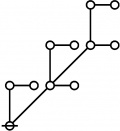$$\text{p} \text{p}_{\text{p} \text{p}_\text{p}}\!$$ $$a(26) ~=~ 3$$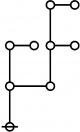$$\text{p}_\text{p}^{\text{p}_\text{p}}\!$$ $$a(27) ~=~ 3$$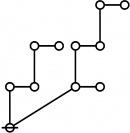$$\text{p}^\text{p} \text{p}_{\text{p}^\text{p}}\!$$ $$a(28) ~=~ 3$$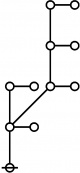$$\text{p}_{\text{p} \text{p}_{\text{p}_\text{p}}}\!$$ $$a(29) ~=~ 4$$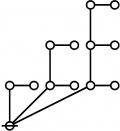$$\text{p} \text{p}_\text{p} \text{p}_{\text{p}_\text{p}}\!$$ $$a(30) ~=~ 3$$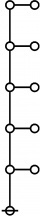$$\text{p}_{\text{p}_{\text{p}_{\text{p}_\text{p}}}}\!$$ $$a(31) ~=~ 5$$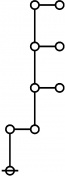$$\text{p}^{\text{p}_{\text{p}_\text{p}}}\!$$ $$a(32) ~=~ 4$$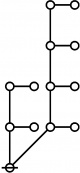$$\text{p}_\text{p} \text{p}_{\text{p}_{\text{p}_\text{p}}}\!$$ $$a(33) ~=~ 4$$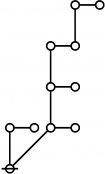$$\text{p} \text{p}_{\text{p}_{\text{p}^\text{p}}}\!$$ $$a(34) ~=~ 4$$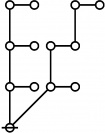$$\text{p}_{\text{p}_\text{p}} \text{p}_{\text{p}^\text{p}}\!$$ $$a(35) ~=~ 3$$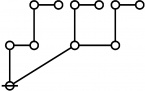$$\text{p}^\text{p} \text{p}_\text{p}^\text{p}\!$$ $$a(36) ~=~ 2$$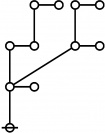$$\text{p}_{\text{p}^\text{p} \text{p}_\text{p}}\!$$ $$a(37) ~=~ 3$$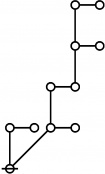$$\text{p} \text{p}_{\text{p}^{\text{p}_\text{p}}}\!$$ $$a(38) ~=~ 4$$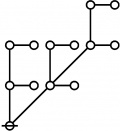$$\text{p}_\text{p} \text{p}_{\text{p} \text{p}_\text{p}}\!$$ $$a(39) ~=~ 3$$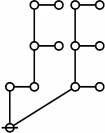$$\text{p}^{\text{p}_\text{p}} \text{p}_{\text{p}_\text{p}}\!$$ $$a(40) ~=~ 3$$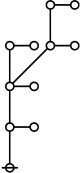$$\text{p}_{\text{p}_{\text{p} \text{p}_\text{p}}}\!$$ $$a(41) ~=~ 4$$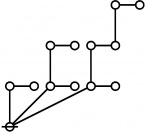$$\text{p} \text{p}_\text{p} \text{p}_{\text{p}^\text{p}}\!$$ $$a(42) ~=~ 3$$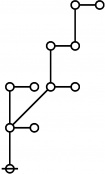$$\text{p}_{\text{p} \text{p}_{\text{p}^\text{p}}}\!$$ $$a(43) ~=~ 4$$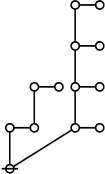$$\text{p}^\text{p} \text{p}_{\text{p}_{\text{p}_\text{p}}}\!$$ $$a(44) ~=~ 4$$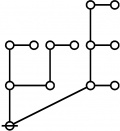$$\text{p}_\text{p}^\text{p} \text{p}_{\text{p}_\text{p}}\!$$ $$a(45) ~=~ 3$$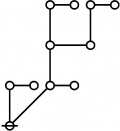$$\text{p} \text{p}_{\text{p}_\text{p}^\text{p}}\!$$ $$a(46) ~=~ 3$$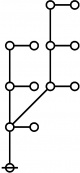$$\text{p}_{\text{p}_\text{p} \text{p}_{\text{p}_\text{p}}}\!$$ $$a(47) ~=~ 4$$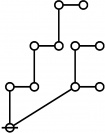$$\text{p}^{\text{p}^\text{p}} \text{p}_\text{p}\!$$ $$a(48) ~=~ 3$$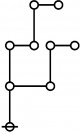$$\text{p}_{\text{p}^\text{p}}^\text{p}\!$$ $$a(49) ~=~ 3$$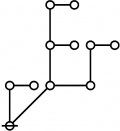$$\text{p} \text{p}_{\text{p}_\text{p}}^\text{p}\!$$ $$a(50) ~=~ 3$$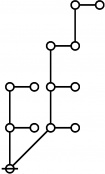$$\text{p}_\text{p} \text{p}_{\text{p}_{\text{p}^\text{p}}}\!$$ $$a(51) ~=~ 4$$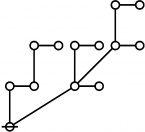$$\text{p}^\text{p} \text{p}_{\text{p} \text{p}_\text{p}}\!$$ $$a(52) ~=~ 3$$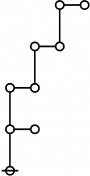$$\text{p}_{\text{p}^{\text{p}^\text{p}}}\!$$ $$a(53) ~=~ 4$$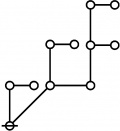$$\text{p} \text{p}_\text{p}^{\text{p}_\text{p}}\!$$ $$a(54) ~=~ 3$$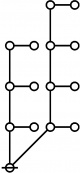$$\text{p}_{\text{p}_\text{p}} \text{p}_{\text{p}_{\text{p}_\text{p}}}\!$$ $$a(55) ~=~ 4$$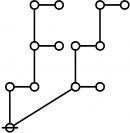$$\text{p}^{\text{p}_\text{p}} \text{p}_{\text{p}^\text{p}}\!$$ $$a(56) ~=~ 3$$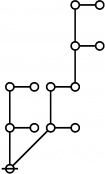$$\text{p}_\text{p} \text{p}_{\text{p}^{\text{p}_\text{p}}}\!$$ $$a(57) ~=~ 4$$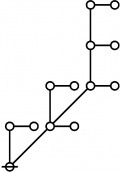$$\text{p} \text{p}_{\text{p} \text{p}_{\text{p}_\text{p}}}\!$$ $$a(58) ~=~ 4$$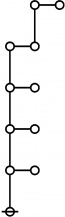$$\text{p}_{\text{p}_{\text{p}_{\text{p}^\text{p}}}}\!$$ $$a(59) ~=~ 5$$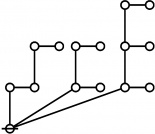$$\text{p}^\text{p} \text{p}_\text{p} \text{p}_{\text{p}_\text{p}}\!$$ $$a(60) ~=~ 3$$